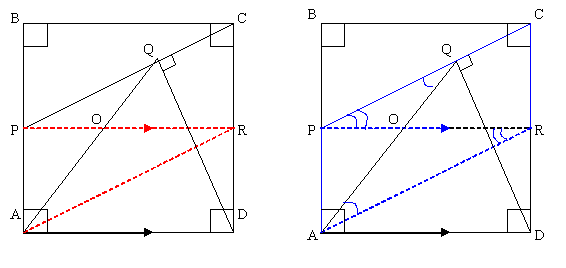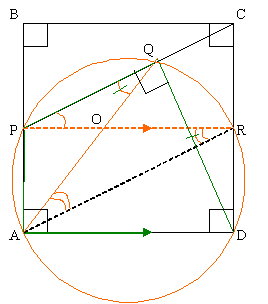#### You may also like### Doodles

Draw a 'doodle' - a closed intersecting curve drawn without taking pencil from paper. What can you prove about the intersections?### Russian Cubes

I want some cubes painted with three blue faces and three red faces. How many different cubes can be painted like that?### Picture Story

Can you see how this picture illustrates the formula for the sum of the first six cube numbers?

# Similarly So

##### Age 14 to 16Challenge Level

Correct and quite different solutions were received from Natasha Kholgade (Indian Language School, Lagos) and Julia Collins (Langley Park School for Girls, England). I have included both of them below. There is a more concise geometrical proof not involving trigonometry for anyone who wants to have another go at this problem but it still depends on the vital insight, noted by Natasha, that APQD is a cyclic quadrilateral.

Natasha's solution:

Given: ABCD is a Square, P is the mid-point of AB and DQ is perpendicular to PC.

Construction: Construct line PR parallel to AD. Join BR.Since BP ll CR and BC ll PR (both ll AD), BPRC is a rectangle and BP = CR Thus AP = BP = CR. Also AP ll CR. Hence APCR is a parallelogram.

Hence PC ll AR. PR is a transversal. Since alternate interior angles are equal,

angleAQP = angleRAQ ?(i)
angleRPQ = angleARP ?(ii)Given angleCQD = 90 o and anglePQD = 90 o . Thus angleCQD + angle PQD = 180 o and PADQ is a cyclic quadrilateral.

Angles in same segment are equal.

Hence angleAQP = angleARP ?(iii)
From (i) and (iii), angleRAQ = angleARP ?(iv)
From (ii) and (iii), angleRPQ = angleAQP ?(v)

Sides opposite equal angles of a triangle are equal.

Hence in triangle POQ, OP = OQ [from (v)] ?(vi)
and in triangleAOR, OR = OA [from (iv)]. ?(vii)

Adding (vi) and (vii), we get

OP + OR = OQ + OA

Or PR = AQ

Since PR ll AD (by construction) and AP ll RD, APRD is a rectangle.

Therefore AD = PR = AQ

Julia's Solution

Let angle $BPC = \alpha$ and the side of the square = $a$

In triangle $BPC$, angle $BCP = 90 - \alpha$

In triangle $QDC$, angle $DCQ = \alpha (90 - \mbox{angle} BCP)$

In triangle $PBC$, $BP = \alpha/2$, $BC = (\alpha\sqrt{5})/2$, $\cos = 1/\sqrt{5}$

In triangle $QCD$,

$QC = a \cos\alpha = a/\sqrt{5}$

$PQ = PC - QC = (a\sqrt5)/2 - a/\sqrt{5} = (3a\sqrt{5})/10$

In triangle $AQD$ - using cosine rule: $$AQ^2 = AP^2 + PQ^2 - 2xAPxPQ \cos(180 - \alpha)$$ $$\quad= a^2/4 + 9a^2/20 - 3a^2\sqrt{5}/10(-\cos\alpha)$$ $$\quad = a^2/4 + 9a^2/20 - 3a^2\sqrt{5}/10(1/\sqrt{5})$$ $$\quad= a^2$$

$AQ = AD = a$

We received the following solution that uses some circle theorems to prove the result:

Angle BPC = angle APD (P is the midpoint of AB, so triangles PAD and PBC are congruent (SAS))

Also, PADQ is a cyclic quadrilateral, because angle PAD and angle CQD are both $90^{\circ}$, and a quadrilateral whose opposite angles add up to $180^{\circ}$ is cyclic.

Thus, angle ADQ = angle BPC, (because angle APQ + angle ADQ add up to $180^{\circ}$, and angles APQ and BPC are on a straight line so also add up to $180^{\circ}$

But angle BPC = angle APD, so angle ADQ = angle APD.
Angle APD and angle AQD are in the same segment so they are equal.

Therefore, triangle AQD is an isosceles triangle, so AQ = AD.